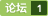### 请问python矩阵叠加操作的问题 [问题点数：40分，无满意结帖，结帖人rocking]Python中的矩阵操作
Numpy 通过观察Python的自有数据类型，我们可以发信啊Python原生并不提供多为数组的<em>操作</em>，那么为了处理<em>矩阵</em>，就需要使用第三方提供的相关的包。 NumPy 是一个非常优秀的提供<em>矩阵</em><em>操作</em>的包。NumPy的主要目标，就是提供多维数组，从而实现<em>矩阵</em><em>操作</em>。 NumPy’s main object is the homogeneous multidimensional array. I...
python+numpy：基本矩阵操作
#! usr/bin/env <em>python</em> # coding: utf-8 # 学习numpy中<em>矩阵</em>的代码笔记 # 2018年05月29日15:43:40 # 参考网站：http://cs231n.github.io/<em>python</em>-numpy-tutorial/ import numpy as np #==================<em>矩阵</em>的创建，增删查改，索引，运算=============...
python矩阵合并、拼接、组合
1 numpy数组 1.1 append() import numpy as np a=np.array([[1,3],[5,7]]) b=np.array([[2,4],[6,8]]) c=np.append(a,b) d=np.append(a,b,axis=0) e=np.append(a,b,axis=1) print("c=\n",c) print("d=\n",d) pr...
Python中矩阵库Numpy基本操作
NumPy是一个关于<em>矩阵</em>运算的库，熟悉Matlab的都应该清楚，这个库就是让<em>python</em>能够进行<em>矩阵</em>话的<em>操作</em>，而不用去写循环<em>操作</em>。下面对numpy中的<em>操作</em>进行总结。 numpy包含两种基本的数据类型：数组和<em>矩阵</em>。数组>>> from numpy import * >>> a1=array([1,1,1]) #定义一个数组 >>> a2=array([2,2,2]) >>> a1+a2
python矩阵合并，增加一行或一列

python将两个二维array叠加成三维array的实现方法
<em>python</em>实现两个三维数组的拼接 <em>python</em>中仅仅依靠调用numpy来实现三维数组的拼接没有对应的函数，但是遇到这样一个需求：每次循环生成一个<em>矩阵</em>，需要把每次循环的<em>矩阵</em><em>叠加</em>成三维<em>矩阵</em>，例如： A=A=A= \$\$ 彩色图片就是三维数组，比如在训练模型时，需要把一批图片都送到网络中就需要把多个三维<em>矩阵</em><em>叠加</em>。...
python矩阵相加函数sum（）
a.sum()是算a中每一元素之和 a.sum(axis=0)是计算a中每一列元素之和 a.sum(axis=1)是计算a中每一行元素之和
python矩阵运算

Python学习（包括：矩阵矩阵的运算，矩阵水平方向和垂直方向的合并，模块化矩阵的序列化和反序列化等）
#coding:utf-8 import numpy as np a =np.array([1,2,3]) b =np.array([4,5,6]) # <em>矩阵</em>的算术运算 必要：两个<em>矩阵</em>的维度相同,运算之后的结果不改变维度 # print(a * b) # 数学计算(线性代数) 两个二维<em>矩阵</em>： 第一个<em>矩阵</em>第一轴 长度 ==第二个<em>矩阵</em>第二个轴长度 a =np.array([[1,2,3],[3,2...
32、python绘制柱形图、单个、多个、堆积、双向柱形图

Python练习题044：矩阵相加

python numpy 矩阵堆叠

python生成矩阵，在矩阵中按行/列插入数据
<em>python</em>用列表生成<em>矩阵</em> arr = [] temp=[] temp.append(1) temp.append(1.234) arr.append(temp) temp1=[] temp1.append(1) temp1.append(1.234) arr.append(temp1) print arr [[1, 1.234], [1, 1.234]] &gt;&gt;&gt; 使用nu...
python3, 计算两个矩形框是否重叠，并计算重叠度
1

Python自动化脚本 for循环+数字拼接
Python自动化脚本 for循环+数字拼接 在写Python自动化脚本时，在执行多个重复<em>操作</em>时遇到的，需将字符串和数字进行拼接，使用for循环，进行<em>操作</em>。
Python合并两个numpy矩阵

python基础练习---矩阵同位置相加问题

Python：合并两个numpy矩阵
numpy是Python用来科学计算的一个非常重要的库，numpy主要用来处理一些<em>矩阵</em>对象，可以说numpy让Python有了Matlab的味道。   如何利用numpy来合并两个<em>矩阵</em>呢？我们可以利用numpy向我们提供的两个函数来进行<em>操作</em>。 #hstack()在行上合并 np.hstack((a,b)) #vstack()在列上合并 np.vstack((a,b)) 以上a，

python——矩阵操作
【<em>问题</em>描述】输入一个N阶<em>矩阵</em>（3&amp;lt;N&amp;lt;10且N为奇数），<em>矩阵</em>中元素均为整数，取值在-1000至+1000之间。将该<em>矩阵</em>中元素的最大值放在<em>矩阵</em>中心，元素的最小值放在<em>矩阵</em>的四个边界上，其余位置换成原<em>矩阵</em>中所有元素的和。 【输入文件】从标准输入读取输入。第一行只有一个整数N，代表<em>矩阵</em>的阶数。 后续有N行输入，每行有N个以若干空格分隔的整数，代表<em>矩阵</em>在该行上的所有元素。 【输出文件】向标准输出...
numpy--拆分以及合并矩阵

numpy中数据拼接的方法
a = np.floor(20 * np.random.random((2,2))) b = np.floor(20 * np.random.random((2,2))) c = np.floor(20 * np.random.random((2,2))) print(a) print(b) print(c) [[ 8. 2.] [16. 15.]] [[ 7. 0.] [10. 12.]]...
Python的矩阵操作
<em>矩阵</em>的生成、计算和与其他类型的转换

#include int main() { int i,j,a,b; int n,m; scanf("%d %d",&n,&m); for(i=0;i<n;i++) for(j=0
Numpy合并矩阵

python如何将多个一维列表进行合并，形成矩阵

Python 矩阵操作
numpy库用于<em>矩阵</em>运算，所以一般先导入它。 import numpy as np 1 <em>矩阵</em>创建 #创建一维数组 a1=array([1,2,3]) #将数组转为<em>矩阵</em> a1=mat(a1) 输出a1：matrix([[1, 2, 3]]) 当然也可以对<em>矩阵</em><em>操作</em>： a1.reshape(3,-1) 这会将<em>矩阵</em>转为3*x的<em>矩阵</em>，因为只有3个元素，所以这里x=1。 其他创建...
Python中numpy矩阵合并和初始化
1、numpy中两个<em>矩阵</em>的合并 1）理论 np.r_[up, down]，把两<em>矩阵</em>上下相加，按列连接两个<em>矩阵</em>，要求列数相等。 np.c_[left, right]，把两<em>矩阵</em>左右相加，按行连接两个<em>矩阵</em>，要求行数相等。 2）例子：     import numpy as np      # up和down都是二维<em>矩阵</em>     up = np.array([[1, 2, 3], [4, 5, 6], [...
Python中多个数组行合并及列合并的几个简单方法

python 矩阵操作 X[:, 0]
# Set min and max values and give it some padding   x_min, x_max = X[:, 0].min() - .5, X[:, 0].max() + .5   y_min, y_max = X[:, 1].min() - .5, X[:, 1].max() + .5 原始数据：<em>矩阵</em> X [[ 1.83145519  0.8939848
numpy合并多维矩阵、list的扩展

python的常见矩阵运算
<em>python</em>的numpy库提供<em>矩阵</em>运算的功能，因此我们在需要<em>矩阵</em>运算的时候，需要导入numpy的包。1.numpy的导入和使用from numpy import *;#导入numpy的库函数 import numpy as np; #这个方式使用numpy的函数时，需要以np.开头。2.<em>矩阵</em>的创建由一维或二维数据创建<em>矩阵</em>from numpy import *; a1=array([1,2,3]);
Python模块学习系列（5）----numy（矩阵部分）

python基础练习（三）—— numpy的矩阵基本操作
<em>python</em>基础练习（三）—— numpy的<em>矩阵</em>基本<em>操作</em>
python numpy 矩阵操作
import numpy np 1. 读写数组，这里可以看成<em>矩阵</em> #返回值格式（评分，信任表，用户个数，项目个数）  a = np.arange(0,12,0.5).reshape(4,-1)  np.savetxt("a.txt", a) # 缺省按照'%.18e'格式保存数据，以空格分隔  np.loadtxt("a.txt")  np.loadtxt('a.txt',dt
python的Numpy之矩阵操作
0.导入numpy包 1.创建<em>矩阵</em> 2.<em>矩阵</em>的基本<em>操作</em> 3.行列式计算 0.导入numpy包 要进行numpy的<em>操作</em>，必须先导入numpy包： import numpy as np 1.创建<em>矩阵</em> 创建<em>矩阵</em>用到 numpy 下的 matrix方法： 如创建<em>矩阵</em>： <em>矩阵</em>行之间用分号间隔 import numpy as np a = np.matrix('1
python矩阵操作小例子

python之numpy对矩阵操作
<em>python</em>的numpy包有很多对<em>矩阵</em>的<em>操作</em>，下面是一些事例：#!/usr/bin/<em>python</em> # -*- coding: UTF-8 -*- # @Time : 17/11/15 下午9:27 # @Author : lijie # @File : mytest06.pyimport numpya1=numpy.array([1,2,3,4]) a2=numpy.array((1,
Python矩阵操作：维度变换、矩阵组合、矩阵分割
1. <em>矩阵</em>维度变换 1.1 numpy.reshape(a, newshape, order=’C’) reshape()函数经常用做一维数组维度的变化，也就是将一维数组变化成为指定维度的<em>矩阵</em>。order是指不同的索引规则，一般默认C，按照行进行运算。 示例： print np.reshape(np.arange(10), (2, 5)) [[0 1 2 3 4] [5 6 7 8...
python中的矩阵操作
1 <em>矩阵</em>相乘例：b1=mat([1,2])#1*2的<em>矩阵</em>b2=mat([,])#2*1的<em>矩阵</em>b3=b1*b2print(b3)2 <em>矩阵</em>点乘(对应元素相乘)例b1=mat([1,2])b2=mat([1,2])b3=multiply(b1,b2)print(b3)3 求逆<em>矩阵</em>例c1=mat(eye(2,2)*0.5)#eye()函数生成对角<em>矩阵</em>，默认对角线为1c2=c1.Iprint(c...
python常见的矩阵运算
<em>python</em>里面经常会用到<em>矩阵</em><em>操作</em>。总结一下：import numpy as np或者使用from numpy import *(用此命令后面<em>操作</em>起来感觉想matlab）类似matlab里面生成各类<em>矩阵</em>一样，这里可以用<em>矩阵</em>相乘：<em>矩阵</em>点乘：<em>矩阵</em>求转置a.T<em>操作</em>，<em>矩阵</em>求逆a.I<em>操作</em>。按列求和和按行求和：求列的最大值索引的位置：argmax(a,0),行的话0改为1<em>矩阵</em>的合并：列：vstack，行：hs...

<em>矩阵</em>在机器学习中算比较常见的，小记一笔最基本的东西。 #-*-coding:utf-8-*- import numpy as np a = np.array([[1,2],[3,4]]) print a b = np.array([[4,5],[6,7]]) print b c= np.array([[-1,1,0],[-4,3,0],[1,0,2]]) print c # 奇异值,这里随便写下,...
【Python】矩阵的拼接操作

python列表相加的方法：两个list [] 加法
<em>python</em> 列表相加可以有两种方法实现： 1 利用<em>操作</em>符+ 比如： a = [1,2,3] b = [4,5,6] c = a+b c的结果：[1,2,3,4,5,6] 2 利用extend 比如： a = [1,2,3] b = [4,5,6] a.extend(b) a的结果：[1,2,3,4,5,6] 结果是一样的，但是+号生成的是一个新的对象，而extend则是在原地的...
python——多个列表对应项求和

numpy数组拼接方法介绍

Python中numpy数组的合并
Python中numpy数组的合并有很多方法，如 - np.append() - np.concatenate() - np.stack() - np.hstack() - np.vstack() - np.dstack() 其中最泛用的是第一个和第二个。第一个可读性好，比较灵活，但是占内存大。第二个则没有内存占用大的<em>问题</em>。
Python科学计算库Numpy里meshgrid用法及矩阵向量合并问题

numpy 合并矩阵

Java学习的正确打开方式

【超详细分析】关于三次握手与四次挥手面试官想考我们什么？

Vue快速实现通用表单验证

2019年Spring Boot面试都问了什么？快看看这22道面试题！
Spring Boot 面试题 1、什么是 Spring Boot？ 2、Spring Boot 有哪些优点？ 3、什么是 JavaConfig？ 4、如何重新加载 Spring Boot 上的更改，而无需重新启动服务器？ 5、Spring Boot 中的监视器是什么？ 6、如何在 Spring Boot 中禁用 Actuator 端点安全性？ 7、如何在自定义端口上运行 Sprin...
Java 数据持久化系列之JDBC

JavaScript 中， 5 种增加代码可读性的最佳实践

Java 9 ← 2017，2019 → Java 13 ，来看看Java两年来的变化

【图解算法面试】记一次面试：说说游戏中的敏感词过滤是如何实现的？

OpenCV-Python 绘图功能 | 七

GitHub 标星 1.6w+，我发现了一个宝藏项目，作为编程新手有福了！

Java知识体系最强总结(2020版)

Windows可谓是大多数人的生产力工具，集娱乐办公于一体，虽然在程序员这个群体中都说苹果是信仰，但是大部分不都是从Windows过来的，而且现在依然有很多的程序员用Windows。 所以，今天我就把我私藏的Windows必装的软件分享给大家，如果有一个你没有用过甚至没有听过，那你就赚了????，这可都是提升你幸福感的高效率生产力工具哦！ 走起！???? NO、1 ScreenToGif 屏幕，摄像头和白板...

Fiddler+夜神模拟器进行APP抓包
Java9到Java13各版本新特性代码全部详解(全网独家原创)
Java现在已经发展到了Java13了（正式版本），相信很多朋友还对各个版本还不是很熟悉，这里面专门把Java9到Java13各个版本的一些新特性做了一些详细讲解。我在网上也找了很多，但基本都是官方文档的CV，没有任何代码演示，而且官方的示例代码也不是很好找得到，官方API目前还是Java10，官方文档真是坑啊。所以我在这里专门写了一篇文章，主要针对平时开发与有关的功能Java9到Java13各...

CPU对每个程序员来说，是个既熟悉又陌生的东西？ 如果你只知道CPU是中央处理器的话，那可能对你并没有什么用，那么作为程序员的我们，必须要搞懂的就是CPU这家伙是如何运行的，尤其要搞懂它里面的寄存器是怎么一回事，因为这将让你从底层明白程序的运行机制。 随我一起，来好好认识下CPU这货吧 把CPU掰开来看 对于CPU来说，我们首先就要搞明白它是怎么回事，也就是它的内部构造，当然，CPU那么牛的一个东...

B 站上有哪些很好的学习资源?

[Pyhon疫情大数据分析] 一.腾讯实时数据爬取、Matplotlib和Seaborn可视化分析全国各地区、某省各城市、新增趋势

2020年十大前沿科技，了解一下

Debugbar+v5.4.1中文版下载